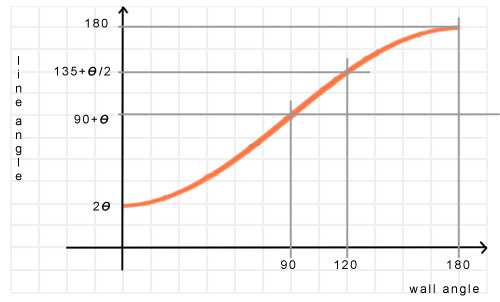BrainDen.com - Brain Teasers

## Question

```Let's say we put up two vertical walls meeting at an 120 degree angle. Then we draw a line on each wall, meeting at the intersection of the walls. Each line makes an angle theta with the horizontal; one goes up from
the intersection and the other goes down. What angle theta should there be that the two lines form the angle 137.5?```

## Recommended Posts

• 0

@bonanova It appears to me that you are way off:

Spoiler

To begin with, we have the angle between the walls fixed at 120 degrees. Label the point of intersection as point O. Measure a distance of 1 unit from point O on each line, and label the points A and B. Draw a horizontal line on each wall through point O. Draw vertical lines through A and B to find points C and D where the vertical lines intersect the horizontal. We now have right triangles ACO and BDO with AO =BO = 1, AC = BD = sin Theta, CO = DO = cos Theta. Note that the midpoint of AB coincides with the midpoint of CD, we'll call this point M. We now have right triangles CMO and AMO with angles COM = 120/2 =60 degrees and AOM = 137.5/2 = 68.75 degrees. We also have right triangle ACM, for which we can find the lengths of each side in terms of Theta.

As stated above, from triangle ACO:

AO = 1 (given)
angle AOC = Theta (given)
angle ACO = 90 (by construction)
AC = sin Theta
CO = cos Theta

From triangle AMO:

angle AOM = 68.75 (bisection of angle AOB, given to be 137.5)
angle AMO = 90 (by construction)
AM = AO * sin AOM = sin 68.75

From triangle CMO:

angle COM = 60 (bisection of angle COD, given to be 120)
angle CMO = 90 (by construction)
CM = CO * sin COM = cos Theta * sin 60

From triangle ACM:

angle ACM = 90 (AC is vertical, CM is horizontal)
AC^2 + CM^2 = AM^2 (pythagorean theorem)

(sin Theta)^2 + (cos Theta * sin 60)^2 = (sin 68.75)^2

(sin Theta)^2 + (cos Theta)^2 * 0.75 = (sin 68.75)^2

(sin Theta)^2 + (cos Theta)^2 * (1 - 0.25) = (sin 68.75)^2

(sin Theta)^2 + (cos Theta)^2 - 0.25 * (cos Theta)^2 = (sin 68.75)^2

1 - 0.25 * (cos Theta)^2 = (sin 68.75)^2

-0.25 * (cos Theta)^2 = (sin 68.75)^2 - 1

0.25 * (cos Theta)^2 = 1 - (sin 68.75)^2

(cos Theta)^2 / 4 = (cos 68.75)^2

(cos Theta) / 2 = cos 68.75

cos Theta = 2 * cos 68.75

Theta = 43.54...

##### Share on other sites

• 0

Here's why:

Spoiler

The angle between the lines (call it Alpha) varies from 2xTheta to 180o as the angle of the walls goes from 0o to 180o. I'm making an educated guess that the variation is a negative cosine function.If so, then at a wall angle of 90o Alpha is midway between the two, namely Theta + 90o. At 120o (an additional 30o) it has increased halfway from there to 180o, an increase of (90o - Theta)/2. So, for a wall angle of 120o we have

Alpha = (90o + Theta) + (90o - Theta)/2 = 135o + Theta/2.

Since we want Alpha to be 137.5o, Theta must be 5o.

##### Share on other sites

• 0

Agreed. Quite a bit off.
Upon further review my guess (of a cosine relationship of line angle to wall angle) was wrong.

Spoiler

Let Alpha be the line angle, omega be the wall angle, and theta be the angle of inclination.
If we follow
Logophobic's analysis and massage things a bit, we get

cos(theta) = cos(alpha/2) / cos(omega/2).

And when omega = 120 this simplifies to

cos(theta) = 2 cos(alpha/2)As Logophobic found, cos(43.541) = 2 cos(68.75).

## Join the conversation

You can post now and register later. If you have an account, sign in now to post with your account.×   Pasted as rich text.   Paste as plain text instead

Only 75 emoji are allowed.

×   Your previous content has been restored.   Clear editor

×   You cannot paste images directly. Upload or insert images from URL.